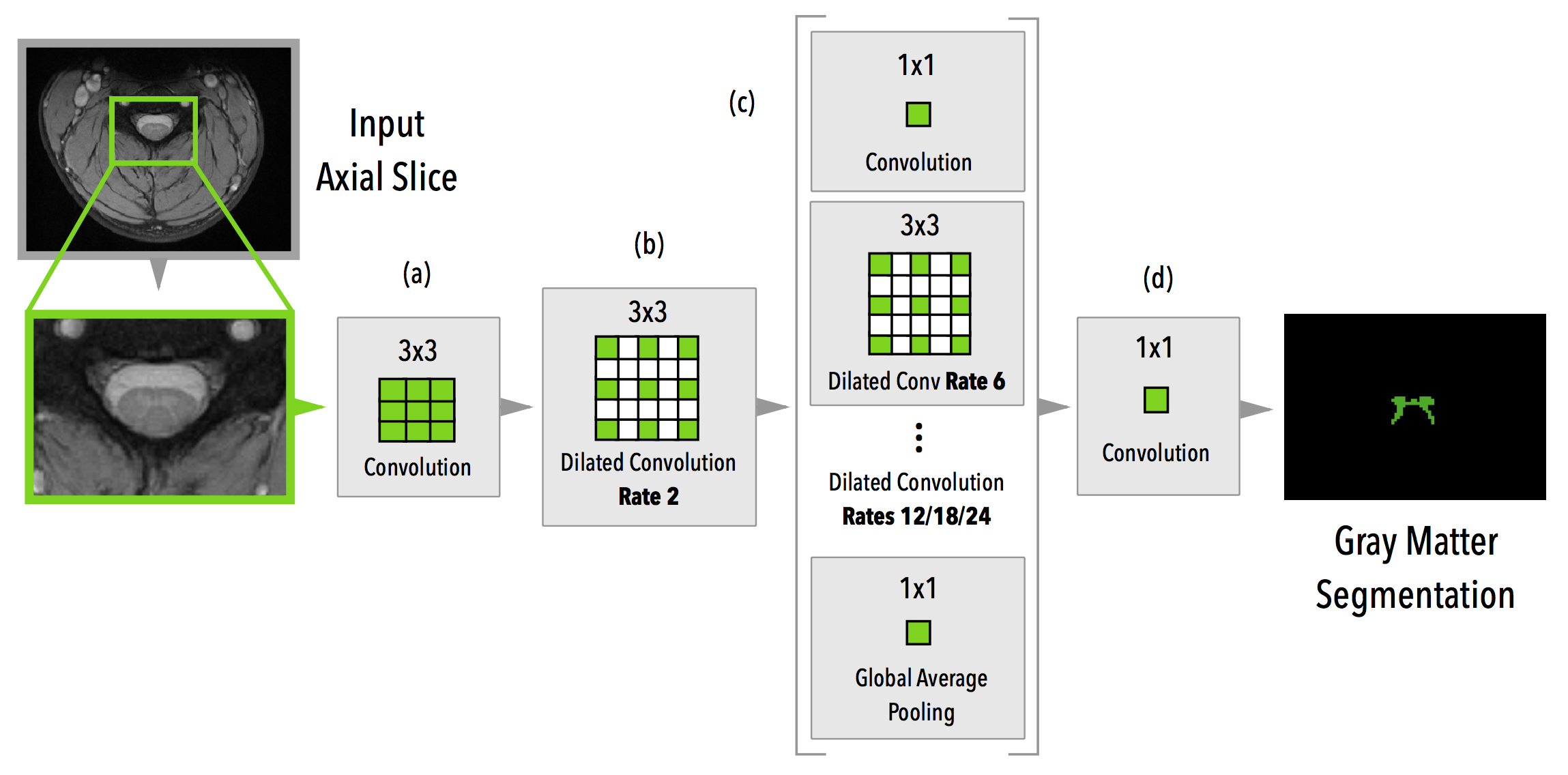# API Documentation¶

All modules above listed are under the “medicaltorch” namespace.

Contents:

## medicaltorch.datasets – Datasets¶

class medicaltorch.datasets.MRI2DSegmentationDataset(filename_pairs, slice_axis=2, cache=True, transform=None, slice_filter_fn=None, canonical=False)[source]

This is a generic class for 2D (slice-wise) segmentation datasets.

Parameters: filename_pairs – a list of tuples in the format (input filename, ground truth filename). slice_axis – axis to make the slicing (default axial). cache – if the data should be cached in memory or not. transform – transformations to apply.
compute_mean_std(verbose=False)[source]

Compute the mean and standard deviation of the entire dataset.

Parameters: verbose – if True, it will show a progress bar. tuple (mean, std dev)
set_transform(transform)[source]

This method will replace the current transformation for the dataset.

Parameters: transform – the new transformation
class medicaltorch.datasets.SCGMChallenge2DTest(root_dir, slice_axis=2, site_ids=None, subj_ids=None, cache=True, transform=None, slice_filter_fn=None, canonical=False)[source]

This is the Spinal Cord Gray Matter Challenge dataset.

Parameters: root_dir – the directory containing the test dataset. site_ids – a list of site ids to filter (i.e. [1, 3]). subj_ids – the list of subject ids to filter. transform – the transformations that should be applied. cache – if the data should be cached in memory or not. slice_axis – axis to make the slicing (default axial).

Note

This dataset assumes that you only have one class in your ground truth mask (w/ 0’s and 1’s). It also doesn’t automatically resample the dataset.

Prados, F., et al (2017). Spinal cord grey matter segmentation challenge. NeuroImage, 152, 312–329. https://doi.org/10.1016/j.neuroimage.2017.03.010

class medicaltorch.datasets.SCGMChallenge2DTrain(root_dir, slice_axis=2, site_ids=None, subj_ids=None, rater_ids=None, cache=True, transform=None, slice_filter_fn=None, canonical=False, labeled=True)[source]

This is the Spinal Cord Gray Matter Challenge dataset.

Parameters: root_dir – the directory containing the training dataset. site_ids – a list of site ids to filter (i.e. [1, 3]). subj_ids – the list of subject ids to filter. rater_ids – the list of the rater ids to filter. transform – the transformations that should be applied. cache – if the data should be cached in memory or not. slice_axis – axis to make the slicing (default axial).

Note

This dataset assumes that you only have one class in your ground truth mask (w/ 0’s and 1’s). It also doesn’t automatically resample the dataset.

Prados, F., et al (2017). Spinal cord grey matter segmentation challenge. NeuroImage, 152, 312–329. https://doi.org/10.1016/j.neuroimage.2017.03.010

class medicaltorch.datasets.SegmentationPair2D(input_filename, gt_filename, cache=True, canonical=False)[source]

This class is used to build 2D segmentation datasets. It represents a pair of of two data volumes (the input data and the ground truth data).

Parameters: input_filename – the input filename (supported by nibabel). gt_filename – the ground-truth filename. cache – if the data should be cached in memory or not. canonical – canonical reordering of the volume axes.
get_pair_data()[source]

Return the tuble (input, ground truth) with the data content in numpy array.

get_pair_shapes()[source]

Return the tuple (input, ground truth) representing both the input and ground truth shapes.

get_pair_slice(slice_index, slice_axis=2)[source]

Return the specified slice from (input, ground truth).

Parameters: slice_index – the slice number. slice_axis – axis to make the slicing.

## medicaltorch.transforms – Transformations¶

class medicaltorch.transforms.CenterCrop2D(size, labeled=True)[source]

Make a center crop of a specified size.

Parameters: segmentation – if it is a segmentation task. When this is True (default), the crop will also be applied to the ground truth.
class medicaltorch.transforms.Normalize(mean, std)[source]

Normalize a tensor image with mean and standard deviation.

Parameters: mean – mean value. std – standard deviation value.
class medicaltorch.transforms.NormalizeInstance[source]

Normalize a tensor image with mean and standard deviation estimated from the sample itself.

Parameters: mean – mean value. std – standard deviation value.
class medicaltorch.transforms.ToTensor(labeled=True)[source]

Convert a PIL image or numpy array to a PyTorch tensor.

## medicaltorch.metrics – Metrics¶

medicaltorch.metrics.numeric_score(prediction, groundtruth)[source]

Computation of statistical numerical scores:

• FP = False Positives
• FN = False Negatives
• TP = True Positives
• TN = True Negatives

return: tuple (FP, FN, TP, TN)

## medicaltorch.models – Models¶

class medicaltorch.models.NoPoolASPP(drop_rate=0.4, bn_momentum=0.1, base_num_filters=64)[source]An ASPP-based model without initial pooling layers.

Parameters: drop_rate – dropout rate. bn_momentum – batch normalization momentum.

Perone, C. S., et al (2017). Spinal cord gray matter segmentation using deep dilated convolutions. Nature Scientific Reports link: https://www.nature.com/articles/s41598-018-24304-3

forward(x)[source]

Model forward pass.

Parameters: x – input data.
class medicaltorch.models.Unet(drop_rate=0.4, bn_momentum=0.1)[source]

A reference U-Net model.

Ronneberger, O., et al (2015). U-Net: Convolutional Networks for Biomedical Image Segmentation ArXiv link: https://arxiv.org/abs/1505.04597

## medicaltorch.losses – Losses¶

class medicaltorch.losses.MaskedDiceLoss(ignore_value=-100.0)[source]

A masked version of the Dice loss.

Parameters: ignore_value – the value to ignore.
medicaltorch.losses.dice_loss(input, target)[source]

Dice loss.

Parameters: input – The input (predicted) target – The target (ground truth) the Dice score between 0 and 1.